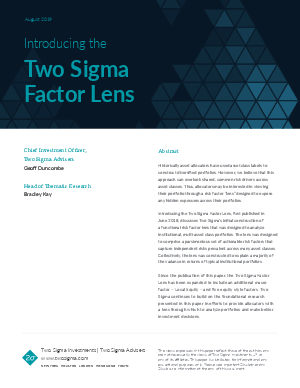> Two Sigma > Introducing the Two Sigma Factor Lens

Introducing the Two Sigma Factor Lens

 Published: Sep 19, 2019 Length: 16 pagesIn this paper, we provide an overview of the Two Sigma Factor Lens, designed for analyzing multi-asset portfolios and derived from returns of broad, liquid asset class proxy indexes. This lens is intended to be:

• Holistic, by capturing the large majority of cross-sectional and time-series risk for typical institutional portfolios;
• Parsimonious, by using as few factors as possible;
• Orthogonal, with each risk factor capturing a statistically uncorrelated risk across assets;
• Actionable, such that desired changes to factor exposure can be readily translated into asset allocation changes.

Finally, we discuss methods for constructing and assessing the Two Sigma Factor Lens that can be extended to produce additional risk factors for new sub-asset-classes or cross-sectional risks that may not currently be captured by the lens. This factor lens, and our ongoing work to expand it, form the foundations of the VennTM platform.

Tags